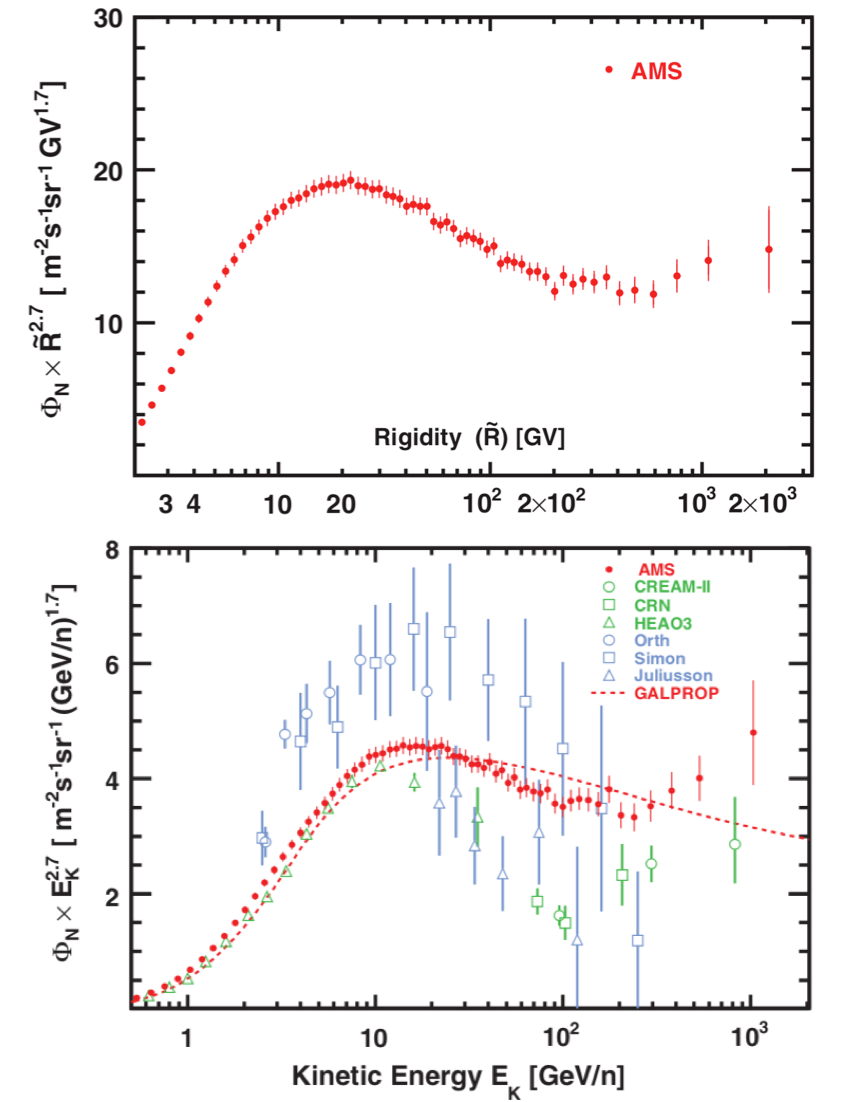# Precision Measurement of Cosmic-Ray Nitrogen and its Primary and Secondary Components

Nitrogen nuclei in cosmic rays are thought to be produced both in astrophysical sources, mostly via the CNO cycle [H. A. Bethe, Phys. Rev. 55, 434 (1939)], and by the collisions of heavier nuclei with the interstellar medium. Therefore, the nitrogen flux $\Phi_{\rm N}$ is expected to contain both primary and secondary components. Precise knowledge of the primary component of cosmic nitrogen provides important insights into the details of nitrogen production in astrophysical sources, while precise knowledge of the secondary component of the cosmic nitrogen provides insights into the details of propagation processes of cosmic rays in the Galaxy.

Over the last 50 years, only a few experiments have measured the nitrogen flux. Typically, these measurements have errors larger than 40%–50% above 100 GV.

AMS published the precise measurement of the nitrogen flux in cosmic rays in the rigidity range from 2.2 GV to 3.3 TV based on 2.2 million nitrogen nuclei collected by AMS during the first five years of operation. The total flux error is 4% at 100 GV. The flux is shown in Figure 1a. The AMS measurement as a function of kinetic energy is presented in Figure 1b in comparison with earlier measurements and with the predictions of cosmic ray propagation model GALPROP. As seen the GALPROP model does not explain our data.Figure 1. (a) The AMS nitrogen flux $\Phi_{\rm N}$ multiplied by $\tilde{R}^{2.7}$with total errors as a function of rigidity. (b) The AMS nitrogen flux $\Phi_{\rm N}$ as a function of kinetic energy per nucleon $E_{K}$ multiplied by $E_{K}^{2.7}$ together with earlier measurements and with the predictions of cosmic ray propagation model GALPROP (dashed red curve).

To determine the primary and secondary components in the nitrogen flux, we have chosen the rigidity dependence of the oxygen flux as characteristic of primary fluxes and the rigidity dependence of the boron flux as characteristic of secondary fluxes. The secondary component of the oxygen flux is the lowest among He, C, and O. The boron flux has no primary contribution and is mostly produced from the interactions of primary cosmic rays C and O with interstellar matter. To obtain the fractions of the primary $\Phi^{P}_{\rm N}$ and secondary $\Phi^{S}_{\rm N}$ components in the nitrogen flux $\Phi_{\rm N}= \Phi^{P}_{\rm N}+\Phi^{S}_{\rm N}$, a fit of $\Phi_{\rm N}$ to the weighted sum of a characteristic primary cosmic ray flux, namely, oxygen $\Phi_{\rm O}$[Observation of identical behavior of He, C and Oxygen  Cosmic Rays at High Rigidities], and of a characteristic secondary cosmic ray flux, namely, boron $\Phi_{\rm B}$ [Observation of New Properties of Secondary Cosmic Rays Lithium, Beryllium and Boron], was performed over the entire rigidity range, as shown in Figure 2. The fit yields $\Phi^{P}_{\rm N} = (0.090\pm0.002) \times \Phi_{\rm O}$ and $\Phi^{S}_{\rm N} = (0.62\pm0.02) \times \Phi_{\rm B}$ with a $\chi^{2}/\mathrm{d.o.f.} = 51/64$.Figure 2. The AMS nitrogen flux $\Phi_{\rm N}$ together with the fit of the weighted sum of the oxygen flux $\Phi_{\rm O}$ and the boron flux $\Phi_{\rm B}$ over the entire rigidity range. The contributions of the primary and secondary components are indicated by the shading (yellow and green, respectively).

Figure 3 shows a) the nitrogen to oxygen (N/O) flux ratio and b) nitrogen to boron (N/B) flux ratio as function of rigidity. As seen, the contribution of the secondary component in the nitrogen flux decreases, and the contribution of the primary component correspondingly increases, with rigidity.Figure 3. a) The AMS $\Phi_{\rm N}/\Phi_{\rm O}$ ratio as a function of rigidity. b) The AMS $\Phi_{\rm N}/\Phi_{\rm B}$ ratio as a function of rigidity. The contributions of the primary and secondary components are indicated by the shading (yellow and green, respectively). As seen from a) and b), the contribution of the secondary component in the nitrogen flux decreases, and the contribution of the primary component correspondingly increases, with rigidity.

The observation that the nitrogen flux can be fit over a wide rigidity range as the simple linear combination of primary and secondary fluxes is a new and important result, which permits the determination of the N/O abundance ratio at the source without the need to consider the Galactic propagation of cosmic rays.  The measured value of N/O abundance at the source of $0.090\pm0.002$ is to be compared to the measured N/O abundance in the Solar system of $0.135_{-0.047}^{+0.051}$ [Synthesis of Elements in Stars, K. Lodders, Springer-Verlag Berlin  Heidelberg p. 379-417 (2010)].

Finally, Figure 4 shows the three distinctly different rigidity dependencies above 30 GV of the primary He, C, and O cosmic ray fluxes, the secondary Li, Be, and B fluxes, and the N flux. As seen, the three secondary fluxes have identical rigidity dependence above 30 GV as do the three primary fluxes above 60 GV, but they are different from each other. The rigidity dependence of the nitrogen flux is different from the dependence of both the primary fluxes and the dependence of the secondary fluxes.Figure 4. Comparison of the AMS measurements of the primary and the secondary cosmic ray fluxes with the nitrogen flux multiplied by $\tilde{R}^{2.7}$ with their total errors as functions of rigidity above 30 GV. For display purposes only, the C, O, Li, Be, B, and N fluxes were rescaled as indicated. For clarity, the horizontal positions of the He, O, Li, and B data points above 400 GV are displaced while C, Be, and N are not.

In conclusion, a precision measurement of the nitrogen flux in cosmic rays from 2.2 GV to 3.3 TV show that, remarkably the nitrogen flux is well described over the entire rigidity range by the sum of the primary flux equal to 9% of the oxygen flux and the secondary flux equal to 62% of the boron flux.